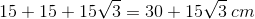# ACT Math : How to find the perimeter of an acute / obtuse isosceles triangle

## Example Questions

### Example Question #215 : Plane Geometry

The height of an isosceles triangle, dropped from the vertex to its base, is one fourth the length of the base. If the area of this triangle is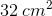, what is its perimeter?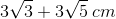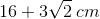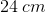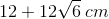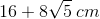Explanation:

Based on the description of this question, you can draw your triangle as such. We can do this thanks to the nature of an isosceles triangle: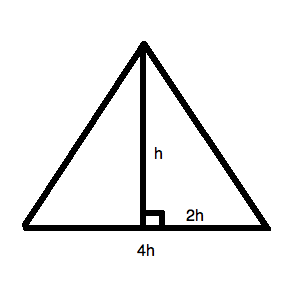Now, you know that the area of a triangle is defined as: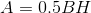So, for our data, we can say: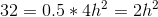Solving for, we get: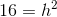Thus,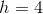.

Now, for our little triangle on the right, we can draw: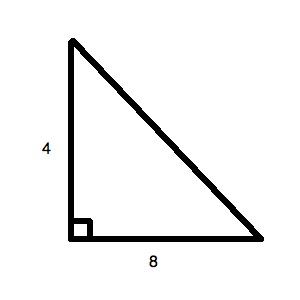Using the Pythagorean Theorem, we know that the other side is: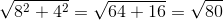This can be simplified to: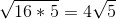Now, we know that this side is the "equal" side of the isosceles triangle. Therefore, we can know that the total perimeter is: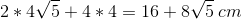### Example Question #216 : Plane Geometry

The base of an isosceles triangle is five times the length of its correlative height. If the area of this triangle is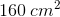, what is its perimeter?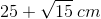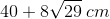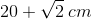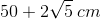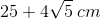Explanation:

Based on the description of this question, you can draw your triangle as such.  We can do this thanks to the nature of an isosceles triangle: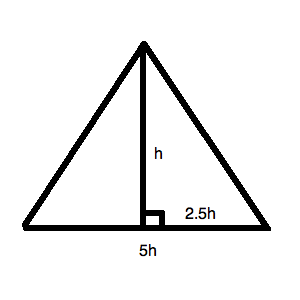Now, you know that the area of a triangle is defined as:So, for our data, we can say: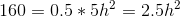Solving for, we get: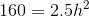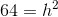Thus,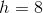.

Now, for our little triangle on the right, we can draw: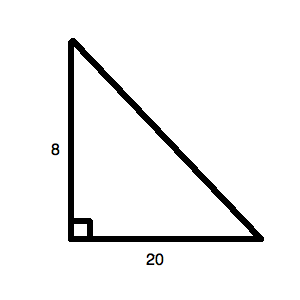Using the Pythagorean Theorem, we know that the other side is: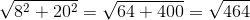This can be simplified to: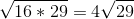Now, we know that this side is the "equal" side of the isosceles triangle. Therefore, we can know that the total perimeter is: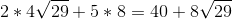### Example Question #1 : Acute / Obtuse Isosceles Triangles

What is the area of an isosceles triangle with a vertex ofdegrees and two sides equal tounits?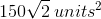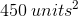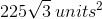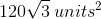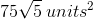Explanation:

Based on the description of your triangle, you can draw the following figure: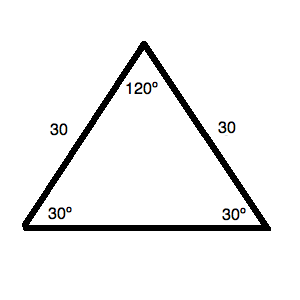You can do this because you know:

1. The two equivalent sides are given.
2. Since a triangle isdegrees, you have only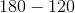ordegrees left for the two angles of equal size. Therefore, those two angles must bedegrees anddegrees.

Now, based on the properties of an isosceles triangle, you can draw the following as well: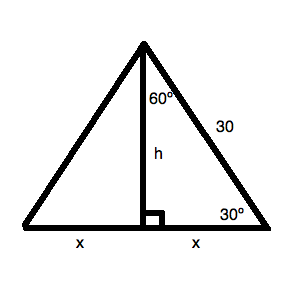Based on your standard reference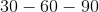triangle, you know: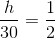Therefore,is.

This means thatis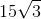, and the total base of the triangle is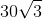.

Now, the area of the triangle is: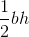or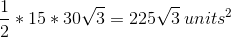### Example Question #218 : Plane Geometry

What is the perimeter of an isosceles triangle with a vertex ofdegrees and two sides equal to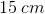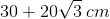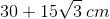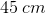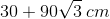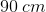Explanation:

Based on the description of your triangle, you can draw the following figure: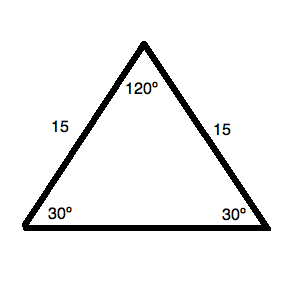You can do this because you know:

1. The two equivalent sides are given.
2. Since a triangle isdegrees, you have onlyordegrees left for the two angles of equal size. Therefore, those two angles must bedegrees anddegrees.

Now, based on the properties of an isosceles triangle, you can draw the following as well: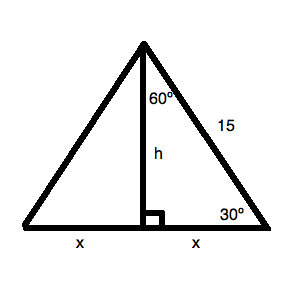Based on your standard referencetriangle, you know: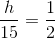Therefore,is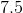.

This means thatis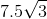and the total base of the triangle is.

Therefore, the perimeter of the triangle is: